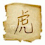## Friday, February 13, 2015

### 52 Things: Number 19: The Shamir secret sharing scheme.

This is the latest in a series of blog posts to address the list of '52 Things Every PhD Student Should Know' to do Cryptography: a set of questions compiled to give PhD candidates a sense of what they should know by the end of their first year.

The Shamir secret sharing scheme , is an algorithm invented by Adi Shamir to allow multiple parties to divide up a secret, such as a key, and when enough of those parts are combined the whole secret can be calculated.

To put it slightly more formally, if we have a secret $S$ and $n$ parties, we can divide $S$ into $n$ parts and distribute those to the various parties. The secret can be divided in such a way that a threshold $k$ can be set so that when $k$ parts of the secret $S$ are known then the whole secret can be calculated. If $k-1$ or less parts of $S$ are known then $S$ can't be calculated. This scheme is called a $(k,n)$ threshold scheme.

The best way to explain how this scheme is constructed is by means of an example. Assume we want to divide the secret $S=1425$ between $5$ parties ($n=5$) and need $3$ parts to be known to allow the secret to be computed. First we construct a polynomial $f(x)$ of order $k-1 = 2$ with random coefficients (say $a_1= 64, a_2 = 112)$ and constant $S$.
$$f(x)= S + a_1 x + a_2 x^2 = 1425 + 64 x + 112 x^2$$
From this polynomial we can construct $n=5$ points, these points can then be distributed between our parties, one point per party.

$P_0=(1,1601),P_1=(2,2001),P_2=(3,2625),P_3=(4,3473),P_4=(5,4545)$

If we assume that we know $3$ of the $5$ points, we can compute $3$ Lagrange Polynomials, the sum of which, when multiplied by the associated $y_j$ values, gives $f(x)$ and therefore gives us the secret $S$. For example if we know
$$(x_0,y_0)=(2,2001), (x_1,y_1)=(3,2625), (x_2,x_3)=(5,4545)$$
Computing 3 Lagrange Polynomials gives
$$l_0 = \frac{x-x_1}{x_0-x_1}\frac{x-x_2}{x_0-x_2} = \frac{1}{3}x^2 - \frac{8}{3}x + 5 \\ l_1 = \frac{x-x_0}{x_1-x_0}\frac{x-x_2}{x_1-x_2} = -\frac{1}{2}x^2 + \frac{7}{2}x - 5 \\ l_2 = \frac{x-x_0}{x_2-x_0}\frac{x-x_1}{x_2-x_1} = \frac{1}{6}x^2 - \frac{5}{6}x + 1 \\ \sum_{j=0}^2 y_j l_j(x) = 112 x^2 + 64 x + 1425.$$
As demonstrated, this method works fine. However, the eavesdropper is able to glean quite a lot of information about the secret $S$; since in the above we have worked with rational arithmetic.However, if we instead work in a finite field (so the secret and the polynomial are defined over a field of size q) then if any two or less parties come together they can learn nothing about the secret.

This is because for two such parties, say party one and party two, and any secret value S' from the field, there is always a degree two polynomial defined over the finite field which interpolates the three values (0,S'), (2,2001 mod q) and (3,2625 mod q).

1.1.